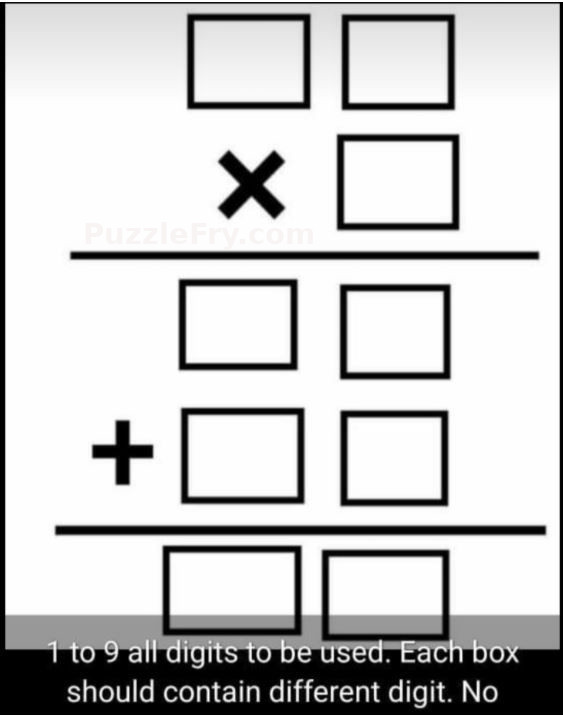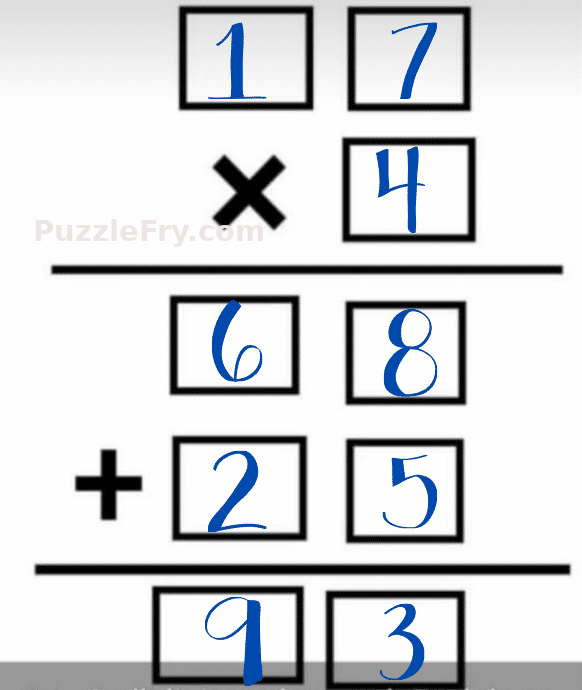# 1 to 9 all digits to be used puzzle

50.3K Views

Use digits from 1 to 9 once in such a way that each digit is used in the boxes and are not repeated.With the help of the mathematical signs, the digits are supposed from the top to bottom and form a mathematical equation where each digit is used only once.Explanation:
As only 1 to 9 all digits need to be used and every box should contain different digit.

If you get such types of puzzles you need to apply and trial method.

In the first two boxes, 1 and 7 will be filled which will get multiplied by 4 i.e. our third box.

17 x 4 = 68

68 will fill the fourth and fifth boxes.

68 + 25 = 93

And then in the sixth and seventh box with 25 which has given result 93 which fills last two boxes.

And no digit got repeated even.

1    7

x      4

_____

= 6   8

+ 2    5

_____

=  9    3

Is it a hit and trial thing or we do have a procedure to solve this puzzle? Please help